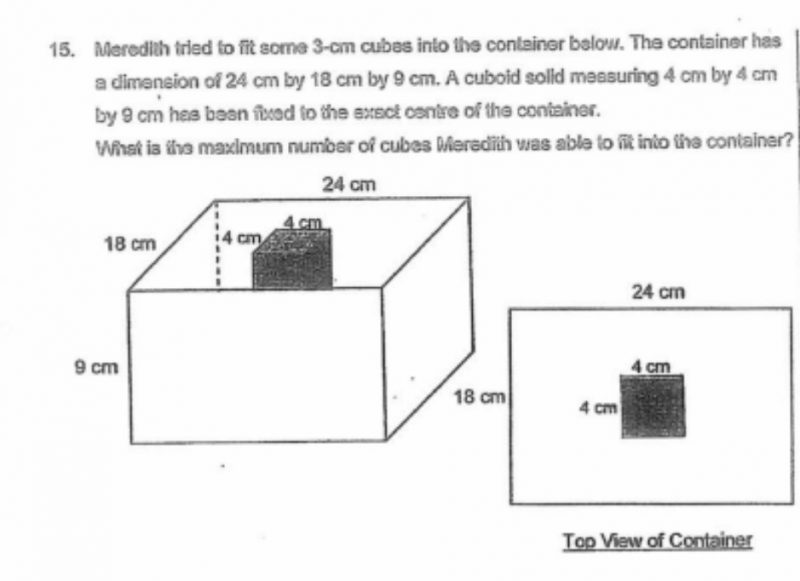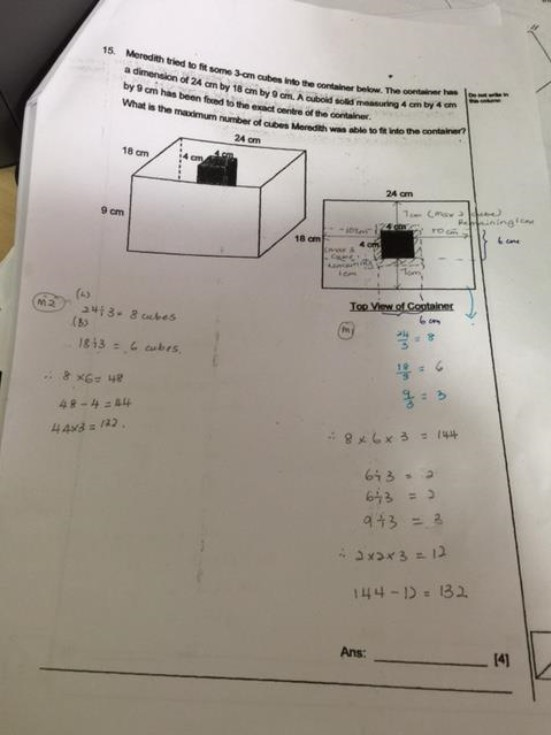# QuestionHave seen workings for this qn but still don’t u/s; especially why need to divide by 2 for some of the answers posted. Need help with a detailed explanation. Thank you v much!

SCGS Prelims 2017 Q15Hi

thank you for working out the qn. Pic not v clear when enlarged. Can take it again?

0 Replies 0 Likes

This is a cuboid question.

Information

1. A cude 4 cm by 4cm has fixed in the centre. Based on the top view, we see Left, Right, Upper, Lower parts.

2. So the left is 10 cm and the right is 10 cm. On the left you can only put 2 cubes, ie 10 ÷ 3 = 3

3. On the right you can put 3 cubes.

4. On the upper part,  you can put 1 cube, ie 7 ÷ 3 = 2.

5. On the lower part, you can put 2 cube only.

 Left Right total R1 3 3 6 R2 2 2 4

6 × 4 = 24 ( 1 layer)

24 × 3 = 72 (3 layers)

😊

Note

This is not a water tank question. Do not find the total volume and divided by the volume of each cube.

0 Replies 1 Like